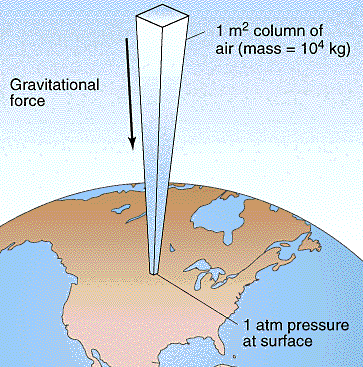# How is air pressure created?

May 1, 2014

The weight of the air above us creates air pressure.

#### Explanation:

Air has weight. The Earth's gravity exerts a force on air molecules, just as it does on us.

The atmosphere extends about 50 km above the Earth's surface, although most of the air is in the lower 20 km.

Assume we could extend a 1 m² column of air 50 km into the atmosphere.The air in this column has a weight of about 10 000 kg. The force of gravity causes this air to exert a pressure on the surface. We call the force exerted per unit of area air pressure.

$F = m a$ = 10 000 kg × 9.8 m•s⁻² = 100 000 kg·m·s⁻²= 100 000 N

$P = \frac{F}{A} = \left(100 000 \text{ N")/(1" m²}\right)$ = 100 000 N·m⁻² = 100 000 Pa = 100 kPa

The weight of the air exerts a pressure of about 100 kPa = 1 atm.